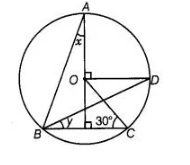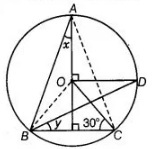# In figure, O is the centre of the`
Question:

In figure, O is the centre of the circle ∠BCO = 30°. Find x and y.Solution:

Given, $O$ is the centre of the circle and $\angle B C O=30^{\circ}$. In the given figure join $O B$ and $A C$.

In $\triangle B O C$,

$C O=B O$      [both are the radius of circle]

$\therefore \quad \angle O B C=\angle O C B=30^{\circ}$

[angles opposite to equal sides are equal]

$\therefore \quad \angle B O C=180^{\circ}-(\angle O B C+\angle O C E)$

[by angle sum property of a triangle]

$=180^{\circ}-\left(30^{\circ}+30^{\circ}\right)=120^{\circ}$

$\angle B O C=2 \angle B A C$We know that, in a circle, the angle subtended by an arc at the centre is twice the angle subtended by it at the remaining part of the circle.

$\therefore \quad \angle B A C=\frac{120^{\circ}}{2}=60^{\circ}$

Also, $\quad \angle B A E=\angle C A E=30^{\circ}$   $[A E$ is an angle bisector of angle $A]$

$\Rightarrow \quad \angle B A E=x=30^{\circ}$

In $\triangle A B E_{1} \quad \angle B A E+\angle E B A+\angle A E B=180^{\circ}$

[by angle sum property of a triangle]

$\Rightarrow \quad 30^{\circ}+\angle E B A+90^{\circ}=180^{\circ}$

$\therefore$ $\angle E B A=180^{\circ}-\left(90^{\circ}+30^{\circ}\right)=180^{\circ}-120^{\circ}=60^{\circ}$

Now, $\angle E B A=60^{\circ}$

$\Rightarrow \quad \angle A B D+y=60^{\circ}$

$\Rightarrow \quad \frac{1}{2} \times \angle A O D+y=60^{\circ}$

[in a circle, the angle subtended by an arc at the centre is twice the angle subtended by it at the remaining part of the circle]

$\Rightarrow \quad \frac{90^{\circ}}{2}+y=60^{\circ} \quad\left[\because \angle \mathrm{AOD}=90^{\circ}\right.$, given $]$

$\Rightarrow \quad 45^{\circ}+y=60^{\circ}$

$\Rightarrow \quad y=60^{\circ}-45^{\circ}$

$\therefore \quad y=15^{\circ}$